8.1 Graphs of the sine and cosine functions  (Page 8/13)

 Page 8 / 13

For the equation $\text{\hspace{0.17em}}A\text{\hspace{0.17em}}\mathrm{cos}\left(Bx+C\right)+D,$ what constants affect the range of the function and how do they affect the range?

The absolute value of the constant $\text{\hspace{0.17em}}A\text{\hspace{0.17em}}$ (amplitude) increases the total range and the constant $\text{\hspace{0.17em}}D\text{\hspace{0.17em}}$ (vertical shift) shifts the graph vertically.

How does the range of a translated sine function relate to the equation $\text{\hspace{0.17em}}y=A\text{\hspace{0.17em}}\mathrm{sin}\left(Bx+C\right)+D?$

How can the unit circle be used to construct the graph of $\text{\hspace{0.17em}}f\left(t\right)=\mathrm{sin}\text{\hspace{0.17em}}t?$

At the point where the terminal side of $\text{\hspace{0.17em}}t\text{\hspace{0.17em}}$ intersects the unit circle, you can determine that the $\text{\hspace{0.17em}}\mathrm{sin}\text{\hspace{0.17em}}t\text{\hspace{0.17em}}$ equals the y -coordinate of the point.

Graphical

For the following exercises, graph two full periods of each function and state the amplitude, period, and midline. State the maximum and minimum y -values and their corresponding x -values on one period for $\text{\hspace{0.17em}}x>0.\text{\hspace{0.17em}}$ Round answers to two decimal places if necessary.

$f\left(x\right)=2\mathrm{sin}\text{\hspace{0.17em}}x$

$f\left(x\right)=\frac{2}{3}\mathrm{cos}\text{\hspace{0.17em}}x$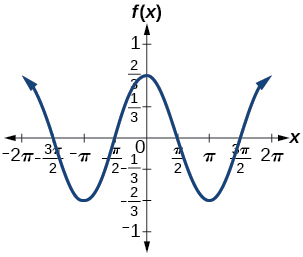amplitude: $\text{\hspace{0.17em}}\frac{2}{3};\text{\hspace{0.17em}}$ period: $\text{\hspace{0.17em}}2\pi ;\text{\hspace{0.17em}}$ midline: $\text{\hspace{0.17em}}y=0;\text{\hspace{0.17em}}$ maximum: $\text{\hspace{0.17em}}y=\frac{2}{3}\text{\hspace{0.17em}}$ occurs at $\text{\hspace{0.17em}}x=0;\text{\hspace{0.17em}}$ minimum: $\text{\hspace{0.17em}}y=-\frac{2}{3}\text{\hspace{0.17em}}$ occurs at $\text{\hspace{0.17em}}x=\pi ;\text{\hspace{0.17em}}$ for one period, the graph starts at 0 and ends at $\text{\hspace{0.17em}}2\pi$

$f\left(x\right)=-3\mathrm{sin}\text{\hspace{0.17em}}x$

$f\left(x\right)=4\mathrm{sin}\text{\hspace{0.17em}}x$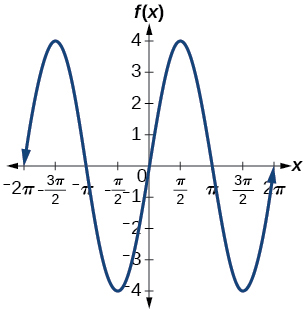amplitude: 4; period: $\text{\hspace{0.17em}}2\pi ;\text{\hspace{0.17em}}$ midline: $\text{\hspace{0.17em}}y=0;\text{\hspace{0.17em}}$ maximum $\text{\hspace{0.17em}}y=4\text{\hspace{0.17em}}$ occurs at $\text{\hspace{0.17em}}x=\frac{\pi }{2};\text{\hspace{0.17em}}$ minimum: $\text{\hspace{0.17em}}y=-4\text{\hspace{0.17em}}$ occurs at $\text{\hspace{0.17em}}x=\frac{3\pi }{2};\text{\hspace{0.17em}}$ one full period occurs from $\text{\hspace{0.17em}}x=0\text{\hspace{0.17em}}$ to $\text{\hspace{0.17em}}x=2\pi$

$f\left(x\right)=2\mathrm{cos}\text{\hspace{0.17em}}x$

$f\left(x\right)=\mathrm{cos}\left(2x\right)$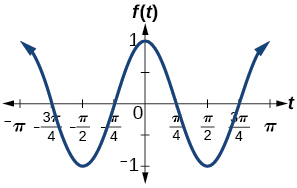amplitude: 1; period: $\text{\hspace{0.17em}}\pi ;\text{\hspace{0.17em}}$ midline: $\text{\hspace{0.17em}}y=0;\text{\hspace{0.17em}}$ maximum: $\text{\hspace{0.17em}}y=1\text{\hspace{0.17em}}$ occurs at $\text{\hspace{0.17em}}x=\pi ;\text{\hspace{0.17em}}$ minimum: $\text{\hspace{0.17em}}y=-1\text{\hspace{0.17em}}$ occurs at $\text{\hspace{0.17em}}x=\frac{\pi }{2};\text{\hspace{0.17em}}$ one full period is graphed from $\text{\hspace{0.17em}}x=0\text{\hspace{0.17em}}$ to $\text{\hspace{0.17em}}x=\pi$

$f\left(x\right)=2\text{\hspace{0.17em}}\mathrm{sin}\left(\frac{1}{2}x\right)$

$f\left(x\right)=4\text{\hspace{0.17em}}\mathrm{cos}\left(\pi x\right)$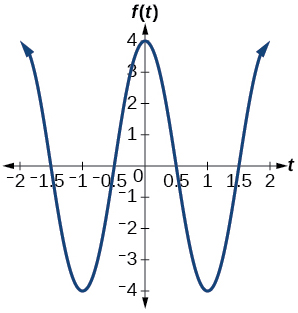amplitude: 4; period: 2; midline: $\text{\hspace{0.17em}}y=0;\text{\hspace{0.17em}}$ maximum: $\text{\hspace{0.17em}}y=4\text{\hspace{0.17em}}$ occurs at $\text{\hspace{0.17em}}x=0;\text{\hspace{0.17em}}$ minimum: $\text{\hspace{0.17em}}y=-4\text{\hspace{0.17em}}$ occurs at $\text{\hspace{0.17em}}x=1$

$f\left(x\right)=3\text{\hspace{0.17em}}\mathrm{cos}\left(\frac{6}{5}x\right)$

$y=3\text{\hspace{0.17em}}\mathrm{sin}\left(8\left(x+4\right)\right)+5$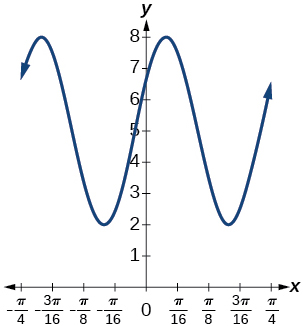amplitude: 3; period: $\text{\hspace{0.17em}}\frac{\pi }{4};\text{\hspace{0.17em}}$ midline: $\text{\hspace{0.17em}}y=5;\text{\hspace{0.17em}}$ maximum: $\text{\hspace{0.17em}}y=8\text{\hspace{0.17em}}$ occurs at $\text{\hspace{0.17em}}x=0.12;\text{\hspace{0.17em}}$ minimum: $\text{\hspace{0.17em}}y=2\text{\hspace{0.17em}}$ occurs at $\text{\hspace{0.17em}}x=0.516;\text{\hspace{0.17em}}$ horizontal shift: $\text{\hspace{0.17em}}-4;\text{\hspace{0.17em}}$ vertical translation 5; one period occurs from $\text{\hspace{0.17em}}x=0\text{\hspace{0.17em}}$ to $\text{\hspace{0.17em}}x=\frac{\pi }{4}$

$y=2\text{\hspace{0.17em}}\mathrm{sin}\left(3x-21\right)+4$

$y=5\text{\hspace{0.17em}}\mathrm{sin}\left(5x+20\right)-2$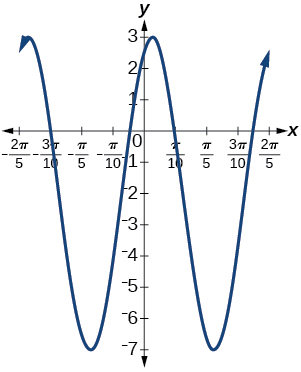amplitude: 5; period: $\text{\hspace{0.17em}}\frac{2\pi }{5};\text{\hspace{0.17em}}$ midline: $\text{\hspace{0.17em}}y=-2;\text{\hspace{0.17em}}$ maximum: $\text{\hspace{0.17em}}y=3\text{\hspace{0.17em}}$ occurs at $\text{\hspace{0.17em}}x=0.08;\text{\hspace{0.17em}}$ minimum: $\text{\hspace{0.17em}}y=-7\text{\hspace{0.17em}}$ occurs at $\text{\hspace{0.17em}}x=0.71;\text{\hspace{0.17em}}$ phase shift: $\text{\hspace{0.17em}}-4;\text{\hspace{0.17em}}$ vertical translation: $\text{\hspace{0.17em}}-2;\text{\hspace{0.17em}}$ one full period can be graphed on $\text{\hspace{0.17em}}x=0\text{\hspace{0.17em}}$ to $\text{\hspace{0.17em}}x=\frac{2\pi }{5}$

For the following exercises, graph one full period of each function, starting at $\text{\hspace{0.17em}}x=0.\text{\hspace{0.17em}}$ For each function, state the amplitude, period, and midline. State the maximum and minimum y -values and their corresponding x -values on one period for $\text{\hspace{0.17em}}x>0.\text{\hspace{0.17em}}$ State the phase shift and vertical translation, if applicable. Round answers to two decimal places if necessary.

$f\left(t\right)=2\mathrm{sin}\left(t-\frac{5\pi }{6}\right)$

$f\left(t\right)=-\mathrm{cos}\left(t+\frac{\pi }{3}\right)+1$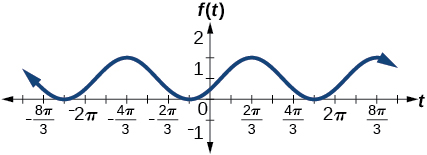amplitude: 1 ; period: $\text{\hspace{0.17em}}2\pi ;\text{\hspace{0.17em}}$ midline: $\text{\hspace{0.17em}}y=1;\text{\hspace{0.17em}}$ maximum: $\text{\hspace{0.17em}}y=2\text{\hspace{0.17em}}$ occurs at $\text{\hspace{0.17em}}x=2.09;\text{\hspace{0.17em}}$ maximum: $\text{\hspace{0.17em}}y=2\text{\hspace{0.17em}}$ occurs at $\text{\hspace{0.17em}}t=2.09;\text{\hspace{0.17em}}$ minimum: $\text{\hspace{0.17em}}y=0\text{\hspace{0.17em}}$ occurs at $\text{\hspace{0.17em}}t=5.24;\text{\hspace{0.17em}}$ phase shift: $\text{\hspace{0.17em}}-\frac{\pi }{3};\text{\hspace{0.17em}}$ vertical translation: 1; one full period is from $\text{\hspace{0.17em}}t=0\text{\hspace{0.17em}}$ to $\text{\hspace{0.17em}}t=2\pi$

$f\left(t\right)=4\mathrm{cos}\left(2\left(t+\frac{\pi }{4}\right)\right)-3$

$f\left(t\right)=-\mathrm{sin}\left(\frac{1}{2}t+\frac{5\pi }{3}\right)$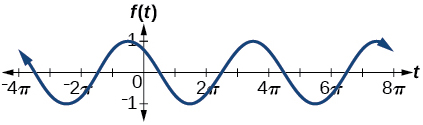amplitude: 1; period: $\text{\hspace{0.17em}}4\pi ;\text{\hspace{0.17em}}$ midline: $\text{\hspace{0.17em}}y=0;\text{\hspace{0.17em}}$ maximum: $\text{\hspace{0.17em}}y=1\text{\hspace{0.17em}}$ occurs at $\text{\hspace{0.17em}}t=11.52;\text{\hspace{0.17em}}$ minimum: $\text{\hspace{0.17em}}y=-1\text{\hspace{0.17em}}$ occurs at $\text{\hspace{0.17em}}t=5.24;\text{\hspace{0.17em}}$ phase shift: $\text{\hspace{0.17em}}-\frac{10\pi }{3};\text{\hspace{0.17em}}$ vertical shift: 0

$f\left(x\right)=4\mathrm{sin}\left(\frac{\pi }{2}\left(x-3\right)\right)+7$

Determine the amplitude, midline, period, and an equation involving the sine function for the graph shown in [link] .

amplitude: 2; midline: $\text{\hspace{0.17em}}y=-3;\text{\hspace{0.17em}}$ period: 4; equation: $\text{\hspace{0.17em}}f\left(x\right)=2\mathrm{sin}\left(\frac{\pi }{2}x\right)-3$

Determine the amplitude, period, midline, and an equation involving cosine for the graph shown in [link] .

Determine the amplitude, period, midline, and an equation involving cosine for the graph shown in [link] .

amplitude: 2; period: 5; midline: $\text{\hspace{0.17em}}y=3;\text{\hspace{0.17em}}$ equation: $\text{\hspace{0.17em}}f\left(x\right)=-2\mathrm{cos}\left(\frac{2\pi }{5}x\right)+3$

Determine the amplitude, period, midline, and an equation involving sine for the graph shown in [link] .

Determine the amplitude, period, midline, and an equation involving cosine for the graph shown in [link] .

amplitude: 4; period: 2; midline: $\text{\hspace{0.17em}}y=0;\text{\hspace{0.17em}}$ equation: $\text{\hspace{0.17em}}f\left(x\right)=-4\mathrm{cos}\left(\pi \left(x-\frac{\pi }{2}\right)\right)$

Determine the amplitude, period, midline, and an equation involving sine for the graph shown in [link] .

Determine the amplitude, period, midline, and an equation involving cosine for the graph shown in [link] .

amplitude: 2; period: 2; midline $\text{\hspace{0.17em}}y=1;\text{\hspace{0.17em}}$ equation: $\text{\hspace{0.17em}}f\left(x\right)=2\mathrm{cos}\left(\pi x\right)+1$

Determine the amplitude, period, midline, and an equation involving sine for the graph shown in [link] .

Algebraic

For the following exercises, let $\text{\hspace{0.17em}}f\left(x\right)=\mathrm{sin}\text{\hspace{0.17em}}x.$

On $\text{\hspace{0.17em}}\left[0,2\pi \right),$ solve $\text{\hspace{0.17em}}f\left(x\right)=0.$

On $\text{\hspace{0.17em}}\left[0,2\pi \right),$ solve $\text{\hspace{0.17em}}f\left(x\right)=\frac{1}{2}.$

$\frac{\pi }{6},\frac{5\pi }{6}$

Evaluate $\text{\hspace{0.17em}}f\left(\frac{\pi }{2}\right).$

On $\text{\hspace{0.17em}}\left[0,2\pi \right),f\left(x\right)=\frac{\sqrt{2}}{2}.\text{\hspace{0.17em}}$ Find all values of $\text{\hspace{0.17em}}x.$

$\frac{\pi }{4},\frac{3\pi }{4}$

On $\text{\hspace{0.17em}}\left[0,2\pi \right),$ the maximum value(s) of the function occur(s) at what x -value(s)?

On $\text{\hspace{0.17em}}\left[0,2\pi \right),$ the minimum value(s) of the function occur(s) at what x -value(s)?

$\frac{3\pi }{2}$

Show that $\text{\hspace{0.17em}}f\left(-x\right)=-f\left(x\right).\text{\hspace{0.17em}}$ This means that $\text{\hspace{0.17em}}f\left(x\right)=\mathrm{sin}\text{\hspace{0.17em}}x\text{\hspace{0.17em}}$ is an odd function and possesses symmetry with respect to ________________.

For the following exercises, let $\text{\hspace{0.17em}}f\left(x\right)=\mathrm{cos}\text{\hspace{0.17em}}x.$

On $\text{\hspace{0.17em}}\left[0,2\pi \right),$ solve the equation $\text{\hspace{0.17em}}f\left(x\right)=\mathrm{cos}\text{\hspace{0.17em}}x=0.$

$\frac{\pi }{2},\frac{3\pi }{2}$

On $\text{\hspace{0.17em}}\left[0,2\pi \right),$ solve $\text{\hspace{0.17em}}f\left(x\right)=\frac{1}{2}.$

On $\text{\hspace{0.17em}}\left[0,2\pi \right),$ find the x -intercepts of $\text{\hspace{0.17em}}f\left(x\right)=\mathrm{cos}\text{\hspace{0.17em}}x.$

$\frac{\pi }{2},\frac{3\pi }{2}$

On $\text{\hspace{0.17em}}\left[0,2\pi \right),$ find the x -values at which the function has a maximum or minimum value.

On $\text{\hspace{0.17em}}\left[0,2\pi \right),$ solve the equation $\text{\hspace{0.17em}}f\left(x\right)=\frac{\sqrt{3}}{2}.$

$\frac{\pi }{6},\frac{11\pi }{6}$

Technology

Graph $\text{\hspace{0.17em}}h\left(x\right)=x+\mathrm{sin}\text{\hspace{0.17em}}x\text{\hspace{0.17em}}$ on $\text{\hspace{0.17em}}\left[0,2\pi \right].\text{\hspace{0.17em}}$ Explain why the graph appears as it does.

Graph $\text{\hspace{0.17em}}h\left(x\right)=x+\mathrm{sin}\text{\hspace{0.17em}}x\text{\hspace{0.17em}}$ on $\text{\hspace{0.17em}}\left[-100,100\right].\text{\hspace{0.17em}}$ Did the graph appear as predicted in the previous exercise?

The graph appears linear. The linear functions dominate the shape of the graph for large values of $\text{\hspace{0.17em}}x.$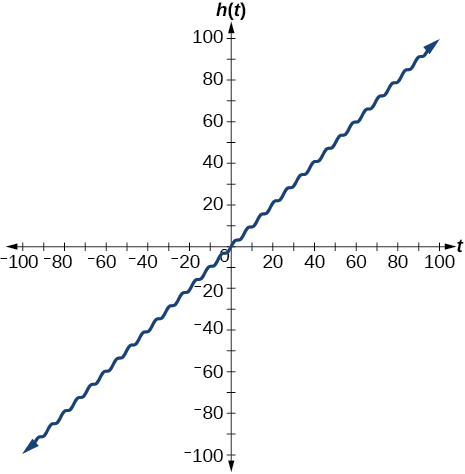Graph $\text{\hspace{0.17em}}f\left(x\right)=x\text{\hspace{0.17em}}\mathrm{sin}\text{\hspace{0.17em}}x\text{\hspace{0.17em}}$ on $\text{\hspace{0.17em}}\left[0,2\pi \right]\text{\hspace{0.17em}}$ and verbalize how the graph varies from the graph of $\text{\hspace{0.17em}}f\left(x\right)=\mathrm{sin}\text{\hspace{0.17em}}x.$

Graph $\text{\hspace{0.17em}}f\left(x\right)=x\text{\hspace{0.17em}}\mathrm{sin}\text{\hspace{0.17em}}x\text{\hspace{0.17em}}$ on the window $\text{\hspace{0.17em}}\left[-10,10\right]\text{\hspace{0.17em}}$ and explain what the graph shows.

The graph is symmetric with respect to the y -axis and there is no amplitude because the function is not periodic.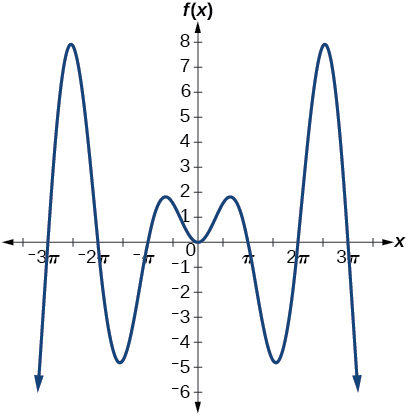Graph $\text{\hspace{0.17em}}f\left(x\right)=\frac{\mathrm{sin}\text{\hspace{0.17em}}x}{x}\text{\hspace{0.17em}}$ on the window $\text{\hspace{0.17em}}\left[-5\pi ,5\pi \right]\text{\hspace{0.17em}}$ and explain what the graph shows.

Real-world applications

A Ferris wheel is 25 meters in diameter and boarded from a platform that is 1 meter above the ground. The six o’clock position on the Ferris wheel is level with the loading platform. The wheel completes 1 full revolution in 10 minutes. The function $\text{\hspace{0.17em}}h\left(t\right)\text{\hspace{0.17em}}$ gives a person’s height in meters above the ground t minutes after the wheel begins to turn.

1. Find the amplitude, midline, and period of $\text{\hspace{0.17em}}h\left(t\right).$
2. Find a formula for the height function $\text{\hspace{0.17em}}h\left(t\right).$
3. How high off the ground is a person after 5 minutes?
1. Amplitude: 12.5; period: 10; midline: $\text{\hspace{0.17em}}y=13.5;$
2. $h\left(t\right)=12.5\mathrm{sin}\left(\frac{\pi }{5}\left(t-2.5\right)\right)+13.5;$
3. 26 ft

sin^4+sin^2=1, prove that tan^2-tan^4+1=0
what is the formula used for this question? "Jamal wants to save \$54,000 for a down payment on a home. How much will he need to invest in an account with 8.2% APR, compounding daily, in order to reach his goal in 5 years?"
i don't need help solving it I just need a memory jogger please.
Kuz
A = P(1 + r/n) ^rt
Dale
how to solve an expression when equal to zero
its a very simple
Kavita
gave your expression then i solve
Kavita
Hy guys, I have a problem when it comes on solving equations and expressions, can you help me 😭😭
Thuli
Tomorrow its an revision on factorising and Simplifying...
Thuli
ok sent the quiz
kurash
send
Kavita
Hi
Masum
What is the value of log-1
Masum
the value of log1=0
Kavita
Log(-1)
Masum
What is the value of i^i
Masum
log -1 is 1.36
kurash
No
Masum
no I m right
Kavita
No sister.
Masum
no I m right
Kavita
tan20°×tan30°×tan45°×tan50°×tan60°×tan70°
jaldi batao
Joju
Find the value of x between 0degree and 360 degree which satisfy the equation 3sinx =tanx
what is sine?
what is the standard form of 1
1×10^0
Akugry
Evalute exponential functions
30
Shani
The sides of a triangle are three consecutive natural number numbers and it's largest angle is twice the smallest one. determine the sides of a triangle
Will be with you shortly
Inkoom
3, 4, 5 principle from geo? sounds like a 90 and 2 45's to me that my answer
Neese
answer is 2, 3, 4
Gaurav
prove that [a+b, b+c, c+a]= 2[a b c]
can't prove
Akugry
i can prove [a+b+b+c+c+a]=2[a+b+c]
this is simple
Akugry
hi
Stormzy
x exposant 4 + 4 x exposant 3 + 8 exposant 2 + 4 x + 1 = 0
x exposent4+4x exposent3+8x exposent2+4x+1=0
HERVE
How can I solve for a domain and a codomains in a given function?
ranges
EDWIN
Thank you I mean range sir.
Oliver
proof for set theory
don't you know?
Inkoom
find to nearest one decimal place of centimeter the length of an arc of circle of radius length 12.5cm and subtending of centeral angle 1.6rad
factoring polynomialByByBy Naveen TomarByBy